# Fisher z-distribution

(Redirected from Fisher-z-distribution(2))The parameters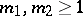are called the degrees of freedom. The characteristic function has the form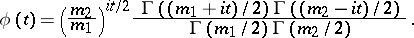The mathematical expectation and the variance are equal to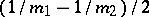and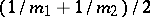, respectively.
If the random variablehas the Fisher-distribution withanddegrees of freedom, then the quantity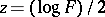has the Fisher-distribution with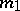and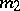degrees of freedom. Along with the Fisher-distribution, known as the distribution of the dispersion proportion, the Fisher-distribution was originally introduced in the analysis of variance by R.A. Fisher (1924). His intention was that the-distribution should be the basic distribution for testing statistical hypotheses in the analysis of variance. The Fisher-distribution was tabulated at the same time, and the first research was concerned with the statistic, although in modern mathematical statistics one uses the simpler statistic.